# Find the equivalent Resistance of the circuit

• Engineering

## Homework Statement

Find the equivalent resistance at terminals a-b of the circuit.

## Homework Equations

Resistors in parallel : 1/Req = 1/R1 + 1/R2...+...1/Rn
Resistors in series: Req = R1 + R2...+...Rn

## The Attempt at a Solution

[/B]
The resistors are in ohms and the answer is 32.5 ohms. The diagram is in the link and so is my work.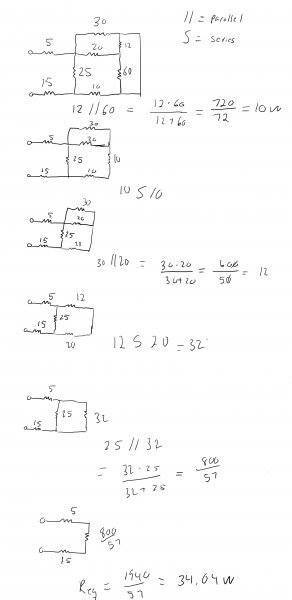gneill
Mentor
In your first step, reducing 12||60 does not leave the 30 in parallel with the 20 as you've shown in the second diagram.

Imagine that you've "shrunk" the wire on the right end, dragging that top node down to the bottom right corner. Where does the 30 Ohm resistor end up connected?

I get 32.5.

[Moderator note: Too much help. Details removed]

The first parallel transformation is the tricky bit. The resulting 10 ohm shares one node with the 20 in the center and the other node it shares with both the 30 and the other 10. If you can visualize the change in topology its easy, if you can't see it try labeling the nodes. After every transformation everything that was connected to each node will be still, the only connections lost will be the inner nodes of a series.
.

Last edited by a moderator:
Merlin3189
Homework Helper
Gold Member
First calculation is correct, but 2nd diagram is wrong.
Try labelling both ends of the parallel resistors (the 12 and the 60) to make sure the result goes in the correct position.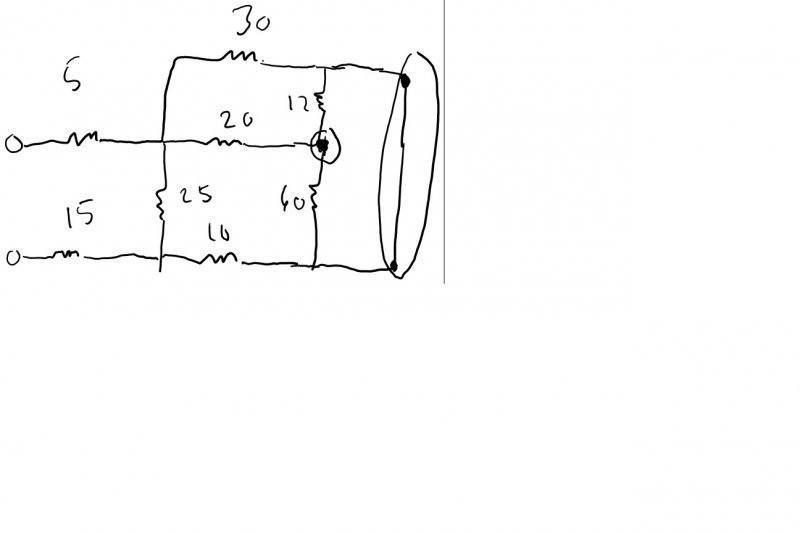I still don't understand how I am supposed to make the diagram after I find the equivalent resistance of the 12 and 60 ohm resistors. I circled their common nodes in this picture but I don't know what to do after for the diagram, sorry.

You didn't circle nodes. Those are corners. Nodes connect components, that is just a bend in a wire.
There are only two nodes on that outer loop that has the 12 and the 60. The center one is easy. Where is the 2nd node?

Merlin3189
Homework Helper
Gold Member
Ok. Try filling in the node table. Each resistor connects to 2 nodes. one at each end.
Remember, lots of wires joined together are still one node. All the components that connect to that wire are going to the same node, because the voltage is the same for all of them where they connect to that wire. Nodes have to be separated by a component.

If you have said that resistors are in parallel, then they must connect to the same two nodes. So their resultant must connect to the same two nodes.

#### Attachments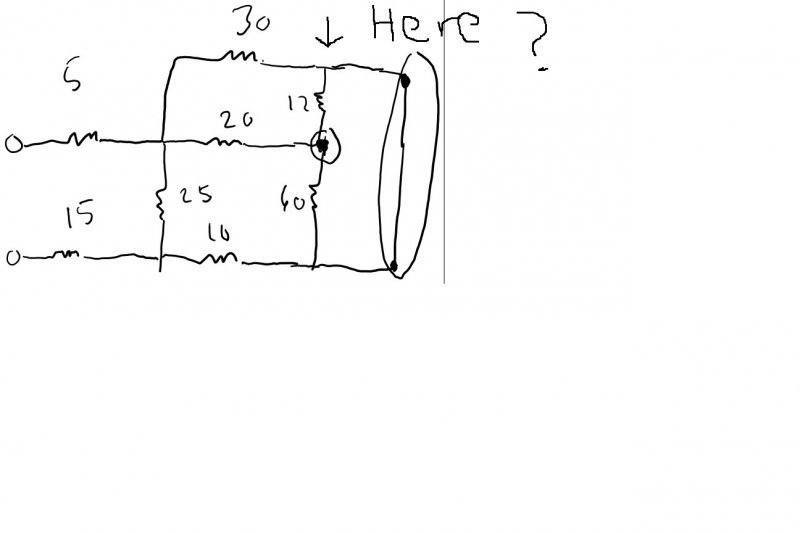Alright I will fill it out. However, wouldn't there also be a node between the 30 ohm resistor and the 12 ohm resistor?

gneill
Mentor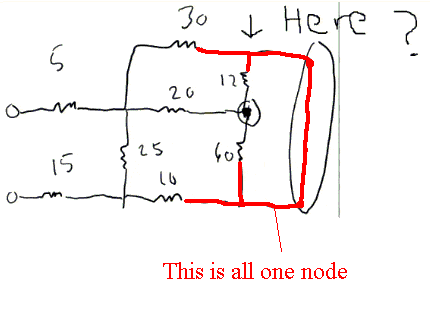A given node comprises all the locations that can be reached by a continuous wire path. It's not just a dot on the circuit diagram.

You are free to rearrange the connection points of components to a given node. As long as the connections remain on the same wire path, the circuit is unchanged. So you can bend and re-route wires, turn components any way you wish, move them to new locations on the page, and so on, so long as the connections remain topologically the same.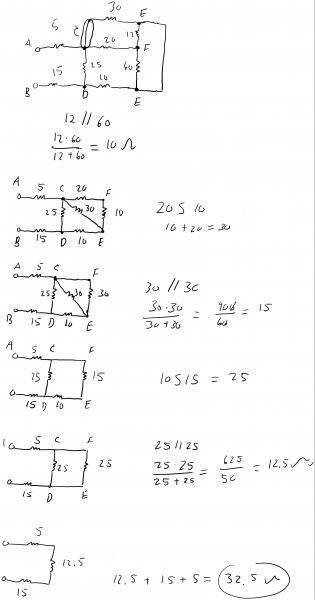Ok this is what I was able to work out.

gneill
Mentor
Yes! Bravo!

Awesome! Thanks guys!

Merlin3189
Homework Helper
Gold Member
Well done. Once you got over that node issue, you were fine.
I do like the way you did a diagram and wrote the calc at each step - just like I do. Some people just write a big math expression and pop it into a calculator. Well, good luck to them, but I like to see what's going on.

Thanks again!